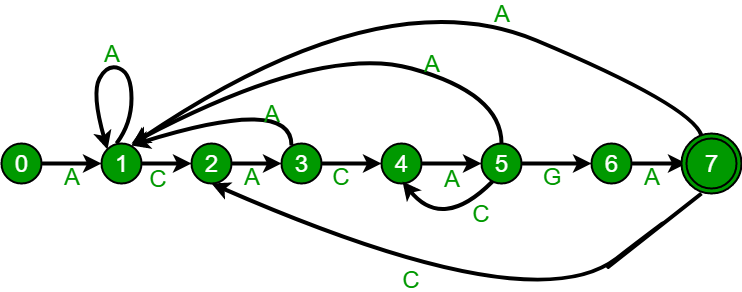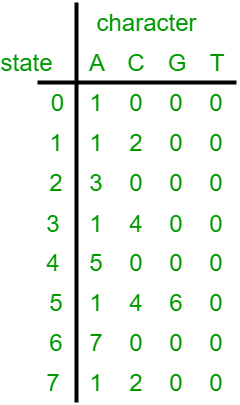Related Articles

# Pattern Searching | Set 6 (Efficient Construction of Finite Automata)

• Difficulty Level : Hard
• Last Updated : 19 Jul, 2021

In the previous post, we discussed Finite Automata based pattern searching algorithm. The FA (Finite Automata) construction method discussed in previous post takes O((m^3)*NO_OF_CHARS) time. FA can be constructed in O(m*NO_OF_CHARS) time. In this post, we will discuss the O(m*NO_OF_CHARS) algorithm for FA construction. The idea is similar to lps (longest prefix suffix) array construction discussed in the KMP algorithm. We use previously filled rows to fill a new row.Attention reader! Don’t stop learning now. Get hold of all the important DSA concepts with the DSA Self Paced Course at a student-friendly price and become industry ready.  To complete your preparation from learning a language to DS Algo and many more,  please refer Complete Interview Preparation Course.

In case you wish to attend live classes with experts, please refer DSA Live Classes for Working Professionals and Competitive Programming Live for Students.The above diagrams represent graphical and tabular representations of pattern ACACAGA.
Algorithm:
1) Fill the first row. All entries in first row are always 0 except the entry for pat character. For pat character, we always need to go to state 1.
2) Initialize lps as 0. lps for the first index is always 0.
3) Do following for rows at index i = 1 to M. (M is the length of the pattern)
…..a) Copy the entries from the row at index equal to lps.
…..b) Update the entry for pat[i] character to i+1.
…..c) Update lps “lps = TF[lps][pat[i]]” where TF is the 2D array which is being constructed.
Following is C/C++ implementation for the above algorithm.
Implementation

## C++

 `#include ``using` `namespace` `std;``#define NO_OF_CHARS 256` `/* This function builds the TF table``which represents Finite Automata for a``given pattern */``void` `computeTransFun(``char``* pat, ``int` `M, ``int` `TF[][NO_OF_CHARS])``{``    ``int` `i, lps = 0, x;` `    ``// Fill entries in first row``    ``for` `(x = 0; x < NO_OF_CHARS; x++)``        ``TF[x] = 0;``    ``TF[pat] = 1;` `    ``// Fill entries in other rows``    ``for` `(i = 1; i <= M; i++) {``        ``// Copy values from row at index lps``        ``for` `(x = 0; x < NO_OF_CHARS; x++)``            ``TF[i][x] = TF[lps][x];` `        ``// Update the entry corresponding to this character``        ``TF[i][pat[i]] = i + 1;` `        ``// Update lps for next row to be filled``        ``if` `(i < M)``            ``lps = TF[lps][pat[i]];``    ``}``}` `/* Prints all occurrences of pat in txt */``void` `search(``char` `pat[], ``char` `txt[])``{``    ``int` `M = ``strlen``(pat);``    ``int` `N = ``strlen``(txt);` `    ``int` `TF[M + 1][NO_OF_CHARS];` `    ``computeTransFun(pat, M, TF);` `    ``// process text over FA.``    ``int` `i, j = 0;``    ``for` `(i = 0; i < N; i++) {``        ``j = TF[j][txt[i]];``        ``if` `(j == M) {``            ``cout << ``"pattern found at index "` `<< i - M + 1 << endl;``        ``}``    ``}``}` `/* Driver code */``int` `main()``{``    ``char` `txt[] = ``"ACACACACAGAAGA ACACAGAACACAGA GEEKS"``;``    ``char` `pat[] = ``"ACACAGA"``;``    ``search(pat, txt);``    ``return` `0;``}` `// This is code is contributed by rathbhupendra`

C

``````
#include <stdio.h>
#include <string.h>
#define NO_OF_CHARS 256

/* This function builds the TF table which represents Finite Automata for a
given pattern  */
void computeTransFun(char* pat, int M, int TF[][NO_OF_CHARS])
{
int i, lps = 0, x;

// Fill entries in first row
for (x = 0; x < NO_OF_CHARS; x++)
TF[x] = 0;
TF[pat] = 1;

// Fill entries in other rows
for (i = 1; i <= M; i++) {
// Copy values from row at index lps
for (x = 0; x < NO_OF_CHARS; x++)
TF[i][x] = TF[lps][x];

// Update the entry corresponding to this character
TF[i][pat[i]] = i + 1;

// Update lps for next row to be filled
if (i < M)
lps = TF[lps][pat[i]];
}
}

/* Prints all occurrences of pat in txt */
void search(char* pat, char* txt)
{
int M = strlen(pat);
int N = strlen(txt);

int TF[M + 1][NO_OF_CHARS];

computeTransFun(pat, M, TF);

// process text over FA.
int i, j = 0;
for (i = 0; i < N; i++) {
j = TF[j][txt[i]];
if (j == M) {
printf("\n pattern found at index %d", i - M + 1);
}
}
}

/* Driver program to test above function */
int main()
{
char* txt = "GEEKS FOR GEEKS";
char* pat = "GEEKS";
search(pat, txt);
getchar();
return 0;
}
``````

## Java

 `/* A Java program to answer queries to check whether``the substrings are palindrome or not efficiently */` `class` `GFG``{` `    ``static` `int` `NO_OF_CHARS = ``256``;` `    ``/* This function builds the TF table``    ``which represents Finite Automata for a``    ``given pattern */``    ``static` `void` `computeTransFun(``char``[] pat,``                                ``int` `M, ``int` `TF[][])``    ``{``        ``int` `i, lps = ``0``, x;` `        ``// Fill entries in first row``        ``for` `(x = ``0``; x < NO_OF_CHARS; x++)``        ``{``            ``TF[``0``][x] = ``0``;``        ``}``        ``TF[``0``][pat[``0``]] = ``1``;` `        ``// Fill entries in other rows``        ``for` `(i = ``1``; i < M; i++)``        ``{``            ``// Copy values from row at index lps``            ``for` `(x = ``0``; x < NO_OF_CHARS; x++)``            ``{``                ``TF[i][x] = TF[lps][x];``            ``}` `            ``// Update the entry corresponding to this character``            ``TF[i][pat[i]] = i + ``1``;` `            ``// Update lps for next row to be filled``            ``if` `(i < M)``            ``{``                ``lps = TF[lps][pat[i]];``            ``}``        ``}``    ``}` `    ``/* Prints all occurrences of pat in txt */``    ``static` `void` `search(``char` `pat[], ``char` `txt[])``    ``{``        ``int` `M = pat.length;``        ``int` `N = txt.length;` `        ``int``[][] TF = ``new` `int``[M + ``1``][NO_OF_CHARS];` `        ``computeTransFun(pat, M, TF);` `        ``// process text over FA.``        ``int` `i, j = ``0``;``        ``for` `(i = ``0``; i < N; i++)``        ``{``            ``j = TF[j][txt[i]];``            ``if` `(j == M)``            ``{``                ``System.out.println(``"pattern found at index "` `+``                                                ``(i - M + ``1``));``            ``}``        ``}``    ``}` `    ``/* Driver code */``    ``public` `static` `void` `main(String[] args)``    ``{``        ``char` `txt[] = ``"GEEKS FOR GEEKS"``.toCharArray();``        ``char` `pat[] = ``"GEEKS"``.toCharArray();``        ``search(pat, txt);``    ``}``}` `// This code is contributed by Princi Singh`

## Python3

 `""" A Python3 program to answer queries to check whether ``the substrings are palindrome or not efficiently """``NO_OF_CHARS ``=` `256` `""" This function builds the TF table``which represents Finite Automata for a``given pattern """`  `def` `computeTransFun(pat, M, TF):` `    ``lps ``=` `0` `    ``# Fill entries in first row``    ``for` `x ``in` `range``(NO_OF_CHARS):``        ``TF[``0``][x] ``=` `0``    ``TF[``0``][``ord``(pat[``0``])] ``=` `1` `    ``# Fill entries in other rows``    ``for` `i ``in` `range``(``1``, M``+``1``):` `        ``# Copy values from row at index lps``        ``for` `x ``in` `range``(NO_OF_CHARS):``            ``TF[i][x] ``=` `TF[lps][x]` `        ``if` `(i < M):``            ``# Update the entry corresponding to this character``            ``TF[i][``ord``(pat[i])] ``=` `i ``+` `1` `            ``# Update lps for next row to be filled` `            ``lps ``=` `TF[lps][``ord``(pat[i])]` `# Prints all occurrences of pat in txt`  `def` `search(pat, txt):``    ``M ``=` `len``(pat)``    ``N ``=` `len``(txt)``    ``TF ``=` `[[``0` `for` `i ``in` `range``(NO_OF_CHARS)] ``for` `j ``in` `range``(M ``+` `1``)]``    ``computeTransFun(pat, M, TF)` `    ``# process text over FA.``    ``j ``=` `0``    ``for` `i ``in` `range``(N):``        ``j ``=` `TF[j][``ord``(txt[i])]``        ``if` `(j ``=``=` `M):``            ``print``(``"pattern found at index"``, i ``-` `M ``+` `1``)`  `# Driver code``txt ``=` `"ACACACACAGAAGA ACACAGAACACAGA GEEKS"``pat ``=` `"ACACAGA"``search(pat, txt)` `# This code is contributed by divyeshrabadiya07`

## C#

 `/* A C# program to answer queries to check whether``the substrings are palindrome or not efficiently */``using` `System;``    ` `class` `GFG``{` `    ``static` `int` `NO_OF_CHARS = 256;` `    ``/* This function builds the TF table``    ``which represents Finite Automata for a``    ``given pattern */``    ``static` `void` `computeTransFun(``char``[] pat,``                                ``int` `M, ``int` `[,]TF)``    ``{``        ``int` `i, lps = 0, x;` `        ``// Fill entries in first row``        ``for` `(x = 0; x < NO_OF_CHARS; x++)``        ``{``            ``TF[0,x] = 0;``        ``}``        ``TF[0,pat] = 1;` `        ``// Fill entries in other rows``        ``for` `(i = 1; i < M; i++)``        ``{``            ``// Copy values from row at index lps``            ``for` `(x = 0; x < NO_OF_CHARS; x++)``            ``{``                ``TF[i,x] = TF[lps,x];``            ``}` `            ``// Update the entry corresponding to this character``            ``TF[i,pat[i]] = i + 1;` `            ``// Update lps for next row to be filled``            ``if` `(i < M)``            ``{``                ``lps = TF[lps,pat[i]];``            ``}``        ``}``    ``}` `    ``/* Prints all occurrences of pat in txt */``    ``static` `void` `search(``char` `[]pat, ``char` `[]txt)``    ``{``        ``int` `M = pat.Length;``        ``int` `N = txt.Length;` `        ``int``[,] TF = ``new` `int``[M + 1,NO_OF_CHARS];` `        ``computeTransFun(pat, M, TF);` `        ``// process text over FA.``        ``int` `i, j = 0;``        ``for` `(i = 0; i < N; i++)``        ``{``            ``j = TF[j,txt[i]];``            ``if` `(j == M)``            ``{``                ``Console.WriteLine(``"pattern found at index "` `+``                                                ``(i - M + 1));``            ``}``        ``}``    ``}` `    ``/* Driver code */``    ``public` `static` `void` `Main(String[] args)``    ``{``        ``char` `[]txt = ``"GEEKS FOR GEEKS"``.ToCharArray();``        ``char` `[]pat = ``"GEEKS"``.ToCharArray();``        ``search(pat, txt);``    ``}``}` `// This code is contributed by Rajput-Ji`

## Javascript

 ``

Output:

``` pattern found at index 0
pattern found at index 10```

Time Complexity for FA construction is O(M*NO_OF_CHARS). The code for search is same as the previous post and time complexity for it is O(n).